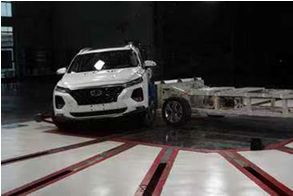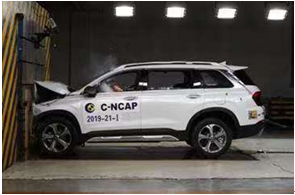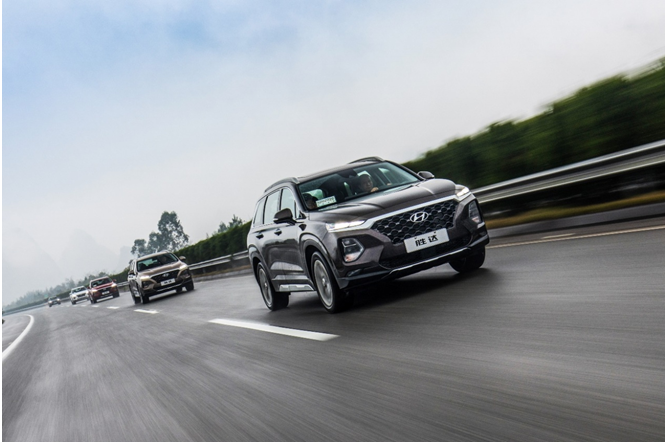## 全家出行的“移动城堡” 北京现代第四代胜达获C-NCAP五星评价

2019年10月11日 17:22 来源：车行天下 超过：13733次关注

日前，中国汽车技术研究中心发布了2019年度第三批C-NCAP评价车型结果。北京现代第四代胜达荣获五星评价，凭借89.6%的得分率力不仅力压全场、超越了本年度参评的其他合资产品，甚至已经逼近奥迪、宝马等豪华车的安全水平。作为一款智享便捷大6座SUV，第四代胜达堪比一座坚固又智能的“移动城堡”，全面保护全家人平安出行。C-NCAP是国内最全面的汽车安全评级机构，历经13年发展、4次规则修订，评价难度不断提升，特别是去年7月开始实施的新版规程更是被称为“史上最严安全评价体系”。北京现代第四代胜达380TGDi GLS 2WD 8AT豪华版参与了本次评价。其在“行人保护”中表现优异，据了解，在我国每年的交通事故中，行人被撞的死亡比例超过40%。拥有优秀的行人保护水平，即使在事故无法避免时，也将对车外行人的伤害降至最低。

新版C-NCAP规程中，对乘员保护的重视程度进行了翻倍的提升。作为一款大6座SUV，第四代胜达面对全家人一起出行的用车场景也能带来出色的安全保障。在本次评价中，第四代胜达“乘员保护”获得了93%的得分率，与以往其他车型只关注前排驾驶员安全的情况相比，第四代胜达实力展现出能充分保障车内每一位家人的出行安全。此外，第四代胜达在“主动安全”评价项目中更是以95.62%的得分率超越同批次其他汽油车型，并最终以89.6%的综合评价得分率领跑本年度合资品牌阵营，不仅远超今年其他批次的日系车型，甚至十分接近奥迪、宝马等德系豪华车的综合评价成绩，当之无愧的荣膺 C-NCAP 五星安全评价，让用户在各种用车场景下都能得到安心的出行保障。能够在全球众多权威测试中屡获大奖，凭借的正是真正能够帮助人们改变生活的“黑科技”。北京现代第四代胜达搭载的Hyundai Smart Sense“智心合一“系统，集成了多达12项主被动安全配置，堪称同级市场中主、被动安全配备最丰富的车型。而安全下车警示功能(SEW)的出现，更是增加了后排儿童开车门时的安全性。在孩子开门瞬间，如果后方恰有车辆驶来，系统就会立即对车内乘客发出警报。哪怕TA学不会“荷式开门法”，也再也不必担心乱开车门导致的交通事故。对于北京现代来说，第四代胜达能够在“史上最严”的C-NCAP新规中仍然摘得五星成绩，不难看出北京现代时刻保持着将国际领先技术导入国内市场，通过不断更新迭代的全新产品为中国消费者带来更安心的出行服务。

#### 相关文章

0-500 字已有评论 0条 查看评论>>

### 热门标签

﻿
• 快速找车
• 选择品牌
• 选择品牌
• A  奥迪
• A  阿斯顿·马丁
• A  阿尔法·罗密欧
• B  宝沃
• B  布加迪
• B  巴博斯
• B  保时捷
• B  宾利
• B  奔驰
• B  宝马
• B  本田
• B  别克
• B  标致
• B  比亚迪
• B  宝骏
• B  北汽制造
• B  北汽新能源
• B  北汽幻速
• B  北汽威旺
• B  北京汽车
• B  奔腾
• B  北汽绅宝
• C  长安
• C  长安商用
• C  长城
• C  昌河
• D  大众
• D  道奇
• D  DS
• D  东南
• D  东风风神
• D  东风风行
• D  东风小康
• D  东风风度
• D  东风
• F  福特
• F  丰田
• F  菲亚特
• F  法拉利
• F  福田
• F  福迪
• F  福汽启腾
• G  观致
• G  广汽传祺
• G  广汽吉奥
• G  GMC
• H  红旗
• H  汉腾汽车
• H  哈弗
• H  哈飞
• H  海格
• H  海马
• H  华颂
• H  黄海
• H  华泰
• H  恒天
• J  吉利汽车
• J  捷豹
• J  Jeep
• J  江淮
• J  江铃
• J  金杯
• J  九龙
• J  金旅
• K  凯翼
• K  凯迪拉克
• K  克莱斯勒
• K  科尼塞克
• K  卡威
• K  开瑞
• L  路虎
• L  林肯
• L  劳斯莱斯
• L  兰博基尼
• L  雷克萨斯
• L  铃木
• L  雷诺
• L  理念
• L  力帆
• L  莲花汽车
• L  猎豹
• L  路特斯
• L  陆风
• M  马自达
• M  MG
• M  MINI
• M  玛莎拉蒂
• M  摩根
• M  迈凯轮
• N  纳智捷
• O  欧宝
• O  讴歌
• O  欧朗
• Q  奇瑞
• Q  起亚
• Q  启辰
• R  日产
• R  荣威
• R  瑞麒
• S  三菱
• S  斯威汽车
• S  萨博
• S  smart
• S  斯柯达
• S  斯巴鲁
• S  思铭
• S  双龙
• S  上汽大通
• S  双环
• T  特斯拉
• T  腾势
• W  沃尔沃
• W  五菱汽车
• W  五十铃
• W  威兹曼
• W  威麟
• X  现代
• X  雪佛兰
• X  雪铁龙
• X  西雅特
• Y  一汽
• Y  英菲尼迪
• Y  英致
• Y  依维柯
• Y  野马汽车
• Y  永源
• Z  众泰
• Z  中华
• Z  中兴
• Z  知豆
• 选择车系
• 选择车系
• 车型对比
• 选择品牌
• 选择品牌
• A  奥迪
• A  阿斯顿·马丁
• A  阿尔法·罗密欧
• B  宝沃
• B  布加迪
• B  巴博斯
• B  保时捷
• B  宾利
• B  奔驰
• B  宝马
• B  本田
• B  别克
• B  标致
• B  比亚迪
• B  宝骏
• B  北汽制造
• B  北汽新能源
• B  北汽幻速
• B  北汽威旺
• B  北京汽车
• B  奔腾
• B  北汽绅宝
• C  长安
• C  长安商用
• C  长城
• C  昌河
• D  大众
• D  道奇
• D  DS
• D  东南
• D  东风风神
• D  东风风行
• D  东风小康
• D  东风风度
• D  东风
• F  福特
• F  丰田
• F  菲亚特
• F  法拉利
• F  福田
• F  福迪
• F  福汽启腾
• G  观致
• G  广汽传祺
• G  广汽吉奥
• G  GMC
• H  红旗
• H  汉腾汽车
• H  哈弗
• H  哈飞
• H  海格
• H  海马
• H  华颂
• H  黄海
• H  华泰
• H  恒天
• J  吉利汽车
• J  捷豹
• J  Jeep
• J  江淮
• J  江铃
• J  金杯
• J  九龙
• J  金旅
• K  凯翼
• K  凯迪拉克
• K  克莱斯勒
• K  科尼塞克
• K  卡威
• K  开瑞
• L  路虎
• L  林肯
• L  劳斯莱斯
• L  兰博基尼
• L  雷克萨斯
• L  铃木
• L  雷诺
• L  理念
• L  力帆
• L  莲花汽车
• L  猎豹
• L  路特斯
• L  陆风
• M  马自达
• M  MG
• M  MINI
• M  玛莎拉蒂
• M  摩根
• M  迈凯轮
• N  纳智捷
• O  欧宝
• O  讴歌
• O  欧朗
• Q  奇瑞
• Q  起亚
• Q  启辰
• R  日产
• R  荣威
• R  瑞麒
• S  三菱
• S  斯威汽车
• S  萨博
• S  smart
• S  斯柯达
• S  斯巴鲁
• S  思铭
• S  双龙
• S  上汽大通
• S  双环
• T  特斯拉
• T  腾势
• W  沃尔沃
• W  五菱汽车
• W  五十铃
• W  威兹曼
• W  威麟
• X  现代
• X  雪佛兰
• X  雪铁龙
• X  西雅特
• Y  一汽
• Y  英菲尼迪
• Y  英致
• Y  依维柯
• Y  野马汽车
• Y  永源
• Z  众泰
• Z  中华
• Z  中兴
• Z  知豆
• 选择车系
• 选择车系
• 选择车型
• 选择车型
• 意见反馈